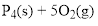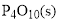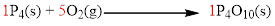# The equation for the oxidation of phosphorus in air is P 4 (s) + 5 O 2 (g) P 4 O 10 (s). Identify the reactants and products and the stoichiometric coefficients. To what do the designations s and g refer?### Chemistry & Chemical Reactivity

10th Edition
John C. Kotz + 3 others
Publisher: Cengage Learning
ISBN: 9781337399074### Chemistry & Chemical Reactivity

10th Edition
John C. Kotz + 3 others
Publisher: Cengage Learning
ISBN: 9781337399074

#### Solutions

Chapter
Section
Chapter 3, Problem 1PS
Textbook Problem

## The equation for the oxidation of phosphorus in air is P4(s) + 5 O2(g)      P4O10(s). Identify the reactants and products and the stoichiometric coefficients. To what do the designations s and g refer?

Expert Solution
Interpretation Introduction

Interpretation:

The reactant, product and stoichiometric coefficient should be identified.  Designation for the g and s should be given.

Concept Introduction:

The law of conservation of mass states that no atoms can be created or destroyed in a chemical reaction, therefore, the number of atoms present in the reactants is equal to the number of atoms present in the products.

The reactant, product and stoichiometric coefficient are given below (a) Designation for the g and s is given below.

Reactant

Phosphorus and oxygenProduct

Tetra phosphorus decaoxideStoichiometric coefficient are 1,5,1.Designation for the g is gas state and s is solid state (b).

### Explanation of Solution

The given equation is shown below using correct molecular formula,In a given reaction left side of the reaction is called reactant and the right side of the reaction is called product. Therefore,

ReactantPhosphorus and oxygen

ProductFor balancing the reaction, stoichiometric coefficient of the reaction is needed therefore the stoichiometric coefficient of the reaction are 1,5,1 and is shown below,Designation for the g is gas state is given below,

g and s state of the constituent.  Therefore g indicates gas state and s indicates solid state.

Conclusion

The reactant, product and stoichiometric coefficient should be identified.  Designation for the g and s were given.

### Want to see more full solutions like this?

Subscribe now to access step-by-step solutions to millions of textbook problems written by subject matter experts!

Get Solutions

### Want to see more full solutions like this?

Subscribe now to access step-by-step solutions to millions of textbook problems written by subject matter experts!

Get Solutions